Ex 6.2

Chapter 6 Class 12 Application of Derivatives
Serial order wise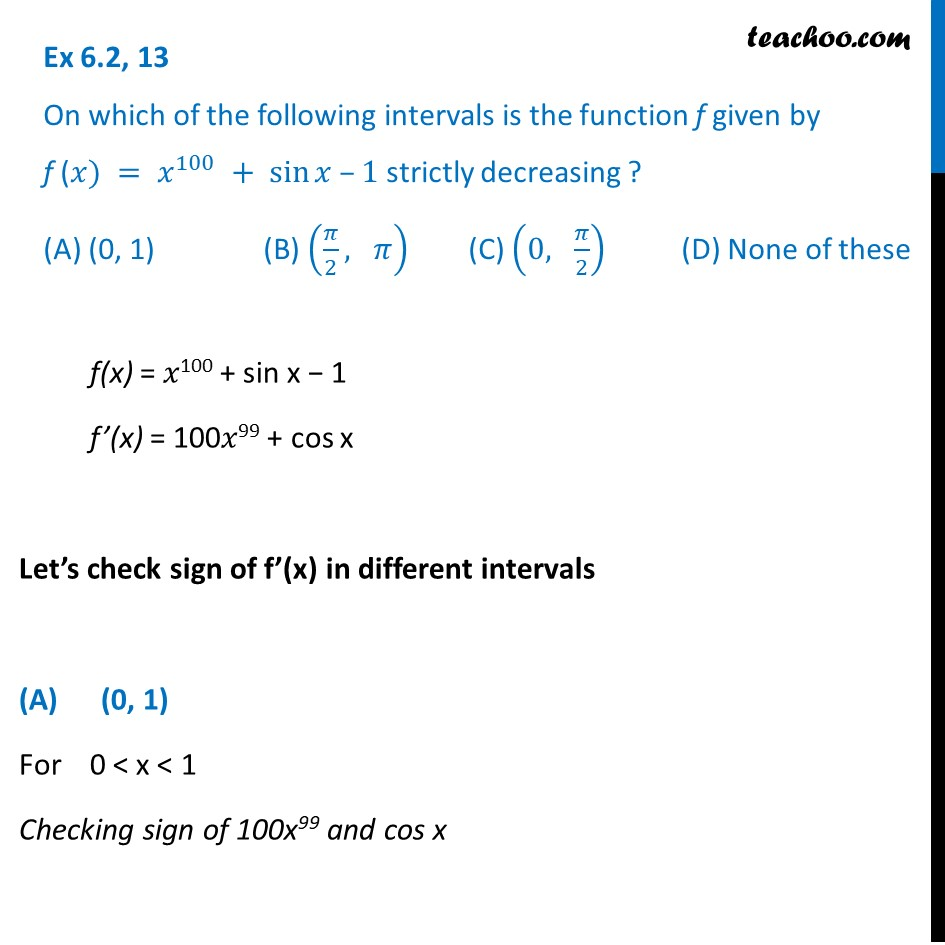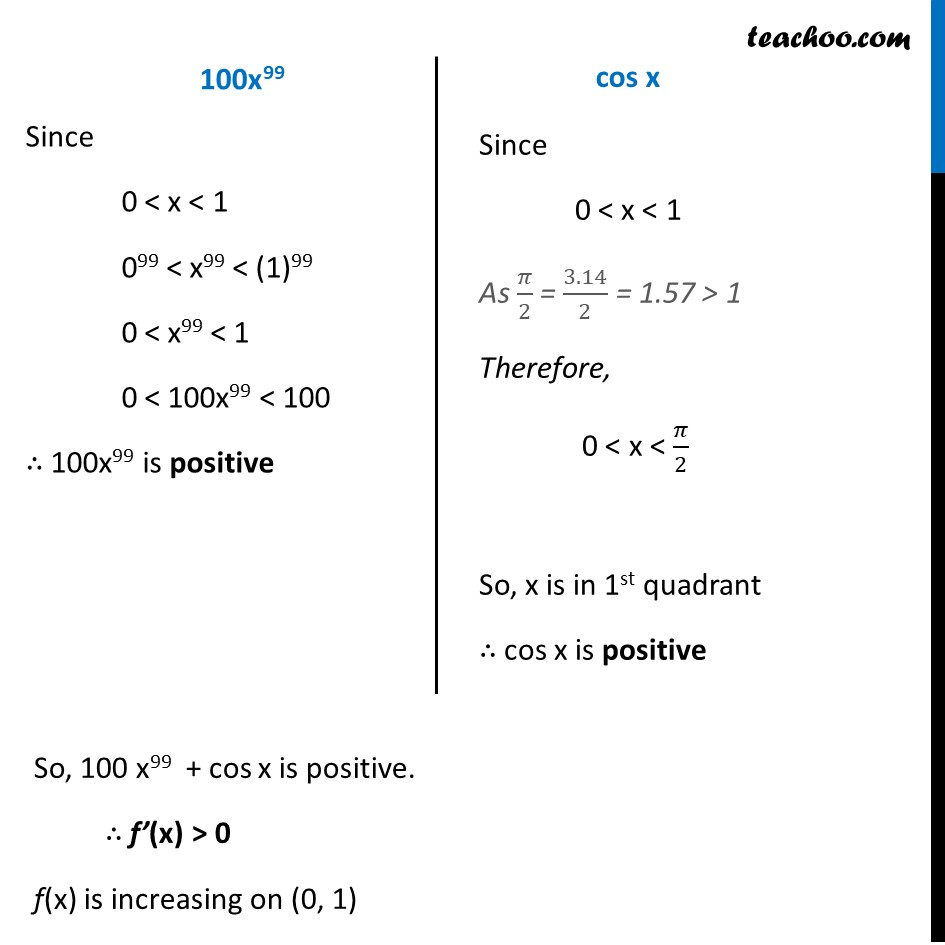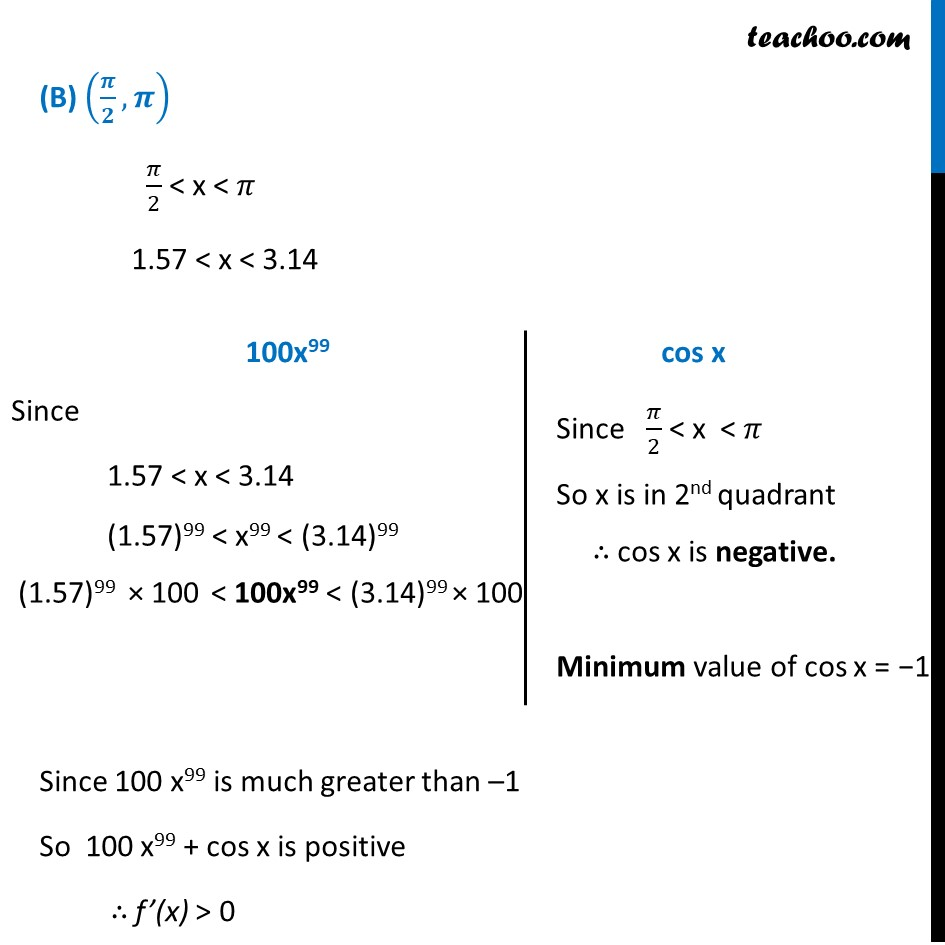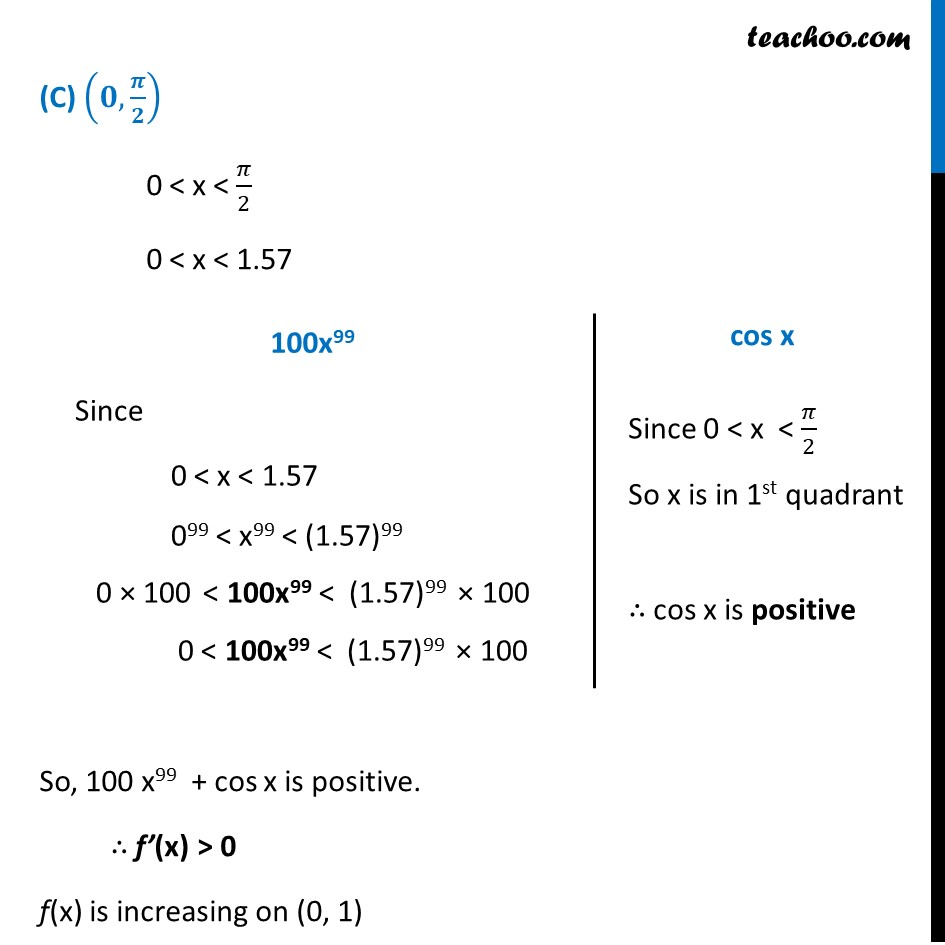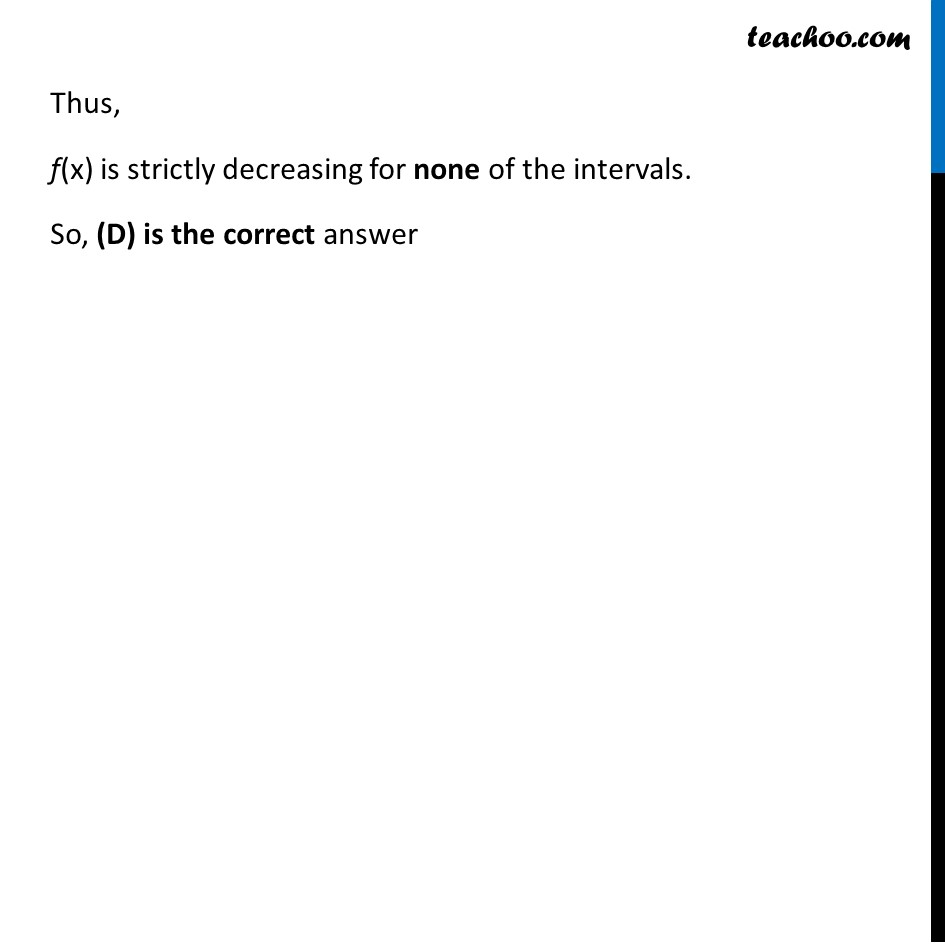Get live Maths 1-on-1 Classs - Class 6 to 12

### Transcript

Ex 6.2, 13 On which of the following intervals is the function f given by f (𝑥) = 𝑥^100 + sin⁡𝑥 –1 strictly decreasing ? (A) (0, 1) (B) (𝜋/2, 𝜋) (C) (0, 𝜋/2) (D) None of these f(x) = 𝑥100 + sin x − 1 f’(x) = 100𝑥99 + cos x Let’s check sign of f’(x) in different intervals (A) (0, 1) For 0 < x < 1 Checking sign of 100x99 and cos x Since 0 < x < 1 099 < x99 < (1)99 0 < x99 < 1 0 < 100x99 < 100 ∴ 100x99 is positive Since 0 < x < 1 As 𝜋/2 = 3.14/2 = 1.57 > 1 Therefore, 0 < x < 𝜋/2 So, x is in 1st quadrant ∴ cos x is positive So, 100 x99 + cos x is positive. ∴ f’(x) > 0 f(x) is increasing on (0, 1) (B) (𝝅/𝟐, 𝝅) 𝜋/2 < x < 𝜋 1.57 < x < 3.14 Since 100 x99 is much greater than –1 So 100 x99 + cos x is positive ∴ f’(x) > 0 Since 1.57 < x < 3.14 (1.57)99 < x99 < (3.14)99 (1.57)99 × 100 < 100x99 < (3.14)99 × 100 Since 𝜋/2 < x < 𝜋 So x is in 2nd quadrant ∴ cos x is negative. Minimum value of cos x = −1 (C) (𝟎,𝝅/𝟐) 0 < x < 𝜋/2 0 < x < 1.57 So, 100 x99 + cos x is positive. ∴ f’(x) > 0 f(x) is increasing on (0, 1) Since 0 < x < 1.57 099 < x99 < (1.57)99 0 × 100 < 100x99 < (1.57)99 × 100 0 < 100x99 < (1.57)99 × 100 Since 0 < x < 𝜋/2 So x is in 1st quadrant ∴ cos x is positive Thus, f(x) is strictly decreasing for none of the intervals. So, (D) is the correct answer